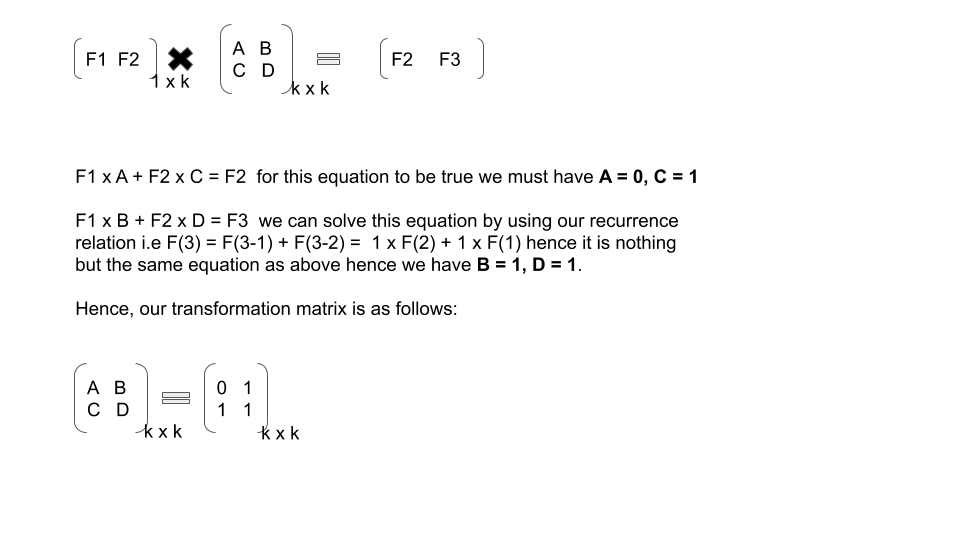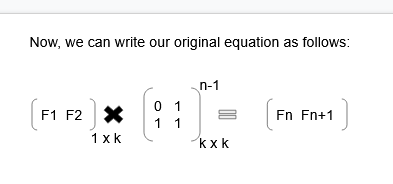## Number Theory - Calculate ‘Nth’ element of recurrence relation in O(logn)

So, after reading our previous article lets continue with our next article on ‘finding Nth element of recurrence relation in O(logn)’.

So, before diving into this article lets understand first what is recurrence relation.

1. What is recurrence relation?

A recurrence relation is an equation that recursively defines a sequence where the next term is a function of the previous terms (ExpressingFn as some combination of Fi with i < n ).

In simple words it is basically an equation that defines a sequence based on a rule that gives the next term as a function of the previous terms.

Example: Fibonacci series – Fn = (Fn – 1) + (Fn – 2) where n > 2

base condition: F(1) = 1
F(2) = 1

We are often required to calculate or solve problems in which we have to form a recurrence relation and then proceed accordingly. But, while dealing with such problems we will definitely be requiring to calculate N th element of our recurrence relation.

So, it becomes necessary to use best possible approach to get N th element of recurrence relation.

2. Naïve Approach (Brute Force):

As you are very much familiar now with what we do in naïve approach, yes we will be simply iterating from 1St term to Nth term and getting our required answer, simple right?

But at the same time notice the time complexity of this approach which is O(n) hence it will definitely lead to Time Limit Exceeded(TLE) in most of the problems.

Don’t worry we will optimize this approach and we will manage to get Accepted 😊

Note : We highly recommend you to try out naïve approach by yourself first and then compare it with our solution, as we are here to make you code better and efficiently.

before looking at implementation it is important to note that we cannot write a general code for every recurrence relation hence we will have to take an example and code for it.

E.g. Fn = (Fn – 1) + (Fn – 2) where n > 2

base condition: F(1) = 1
F(2) = 1

Below is the implementation of naïve approach for above recurrence relation:

Time Complexity: O(n)

3. Optimized Approach – O(logn):

So, After looking at brute force approach we will try to optimize our approach with some of our observation.

let’s understand our observation with a simple example:

E.g. Fn = (Fn – 1) + (Fn – 2) where n > 2

base condition : F(1) = 1
F(2) = 1

Below is the step by step process to proceed with optimization:

Step1: Firstly, determine the number of elements on which our current term (i.e F­­n ) of recurrence relation depends ( i.e. K). K = 2, in our case.

Step2: Determine base values or initial values (i.e. F(1) = 1, F(2) = 1 in our case)

Step3: Determine Transformation matrix: The transformation matrix in this context is a special matrix with which we will multiply our initial values matrix and based upon the power of transformation matrix we will get terms of our recurrence relation.

The dimension of transformation matrix is taken as K x K.

Note: here the power of transformation matrix is calculated using Matrix Exponentiation, if you want to know more about it click here.

You can visualize this process as below:

Consider F1 and F2 as our initial terms and since in our case value of k = 2, we will be using a 2 x 2 matrix as a transformation matrix.

Note: Below written equation is true for every linear recurrence relation.Now, we have got our final equation to calculate Nth element of recurrence relation.

Note: here the power of transformation matrix is calculated using Matrix Exponentiation, if you want to know more about it click here.

Below is the implementation of optimized approach for above recurrence relation:

Time Complexity: O(logn)

It is always better to solve a question after you learn a new concept as it helps in making concrete concepts.

So, below are problems which are handpicked by Programmers Army to make your concept more solid which you learned.

1. Problem from Programmers Army: Try writing code for finding Nth term of this recurrence relation F(n) = 2Fn-1 + 3Fn-2   for n > 2
F(1) = 2, F(2) = 3;